# Analog_Rotation_Sensor_V2__SKU__DFR0058_-DFRobot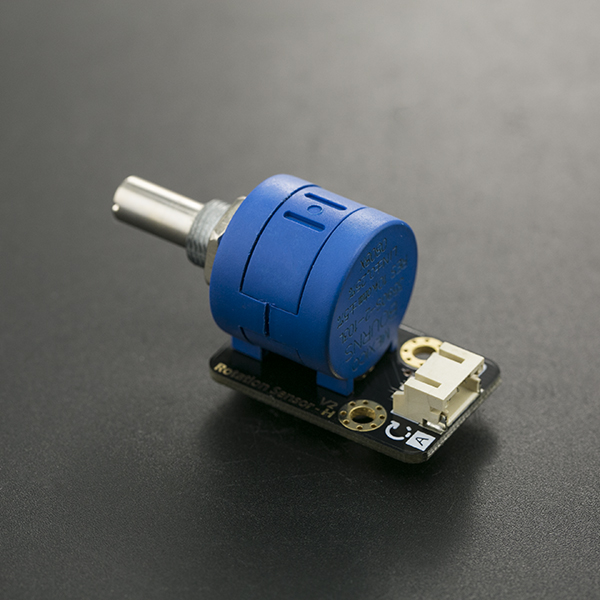## Introduction

This V2 Rotation Sensor is arduino compatible multi-ring rotation analog sensor. It is based on multi-turn precision potentiometer. It can rotate upto 10 laps. If the sensor input 5V voltage power, then the 5000mV will be divided into 3600 portions(10 laps), when you adjust the rotation of the 3 degrees and then the voltage will have 2mV change, so you can accurately achieve the effect of voltage with small changes. and it is easy to be connected to Arduino or DFRduino with our sensor expansion board.

## Specification

• Arduino compatible
• Operating Voltage: +3.3-5V DC
• Signal Type: analog signal
• Size: 50x22mm
• Tnterface Mode: Gravity interface (PH2.0-3)
• Rotation Angle: 3600 degrees
• Weight: 20g

## Pin Definition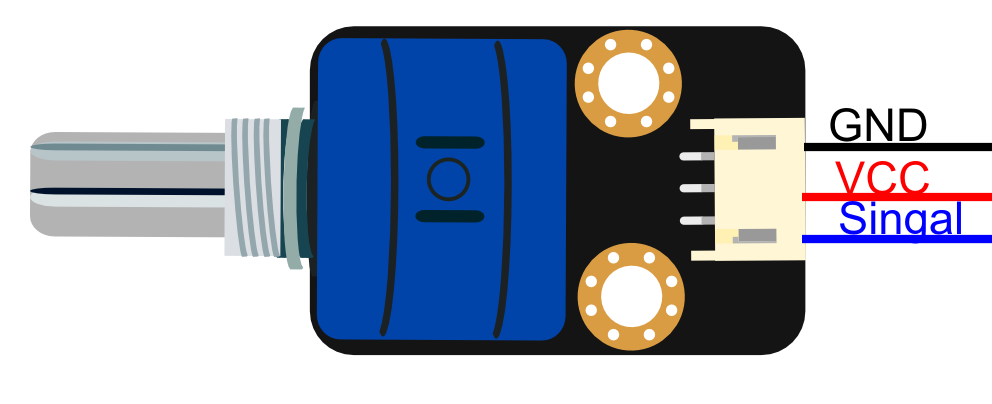Color Description
BLACK GND
RED VCC
BLUE Analog Signal Output

## Tutorial

In this tutorial we rotate the Analog Rotation Sensor V2 to output the analog voltage value in the serial port.

### Connection Diagram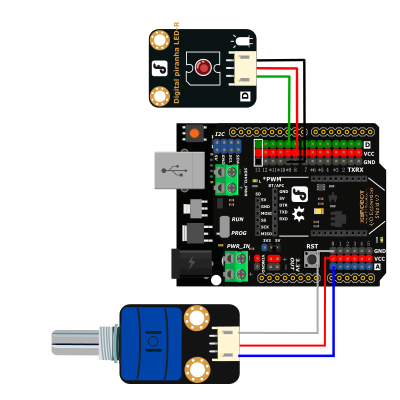### Sample Code

``````const int analogInPin = A0;  // Analog input pin that the potentiometer is attached to
const int analogOutPin = 9; // Analog output pin that the LED is attached to

int sensorValue = 0;        // value read from the pot
int outputValue = 0;        // value output to the PWM (analog out)

void setup() {
// initialize serial communications at 9600 bps:
Serial.begin(9600);
}

void loop() {
// map it to the range of the analog out:
outputValue = map(sensorValue, 0, 1023, 0, 255);
// change the analog out value:
analogWrite(analogOutPin, outputValue);

// print the results to the serial monitor:
Serial.print("sensor = ");
Serial.print(sensorValue);
Serial.print("\t output = ");
Serial.println(outputValue);

// wait 2 milliseconds before the next loop
// for the analog-to-digital converter to settle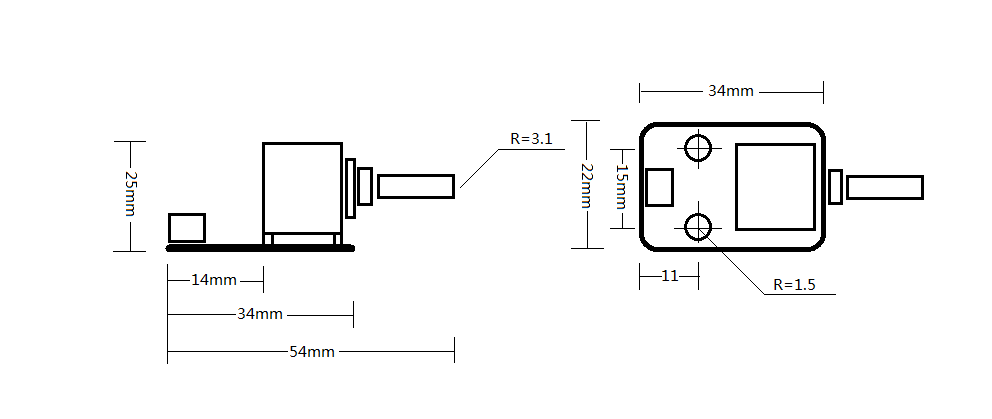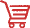Get Gravity: Analog Rotation Potentiometer Sensor V2 For Arduino from DFRobot Store or DFRobot Distributor.